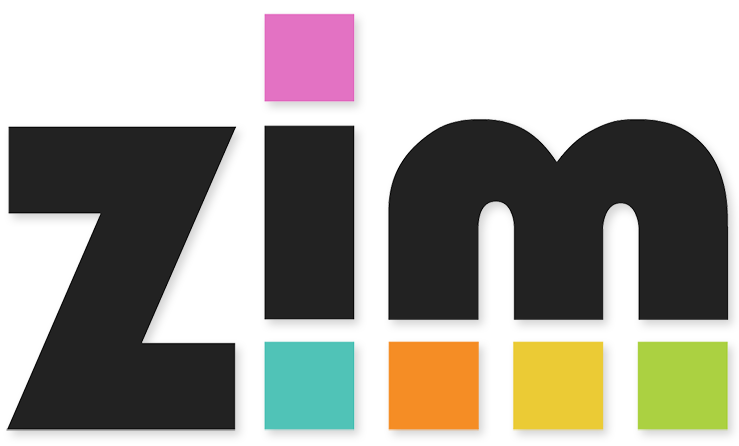## Handy Counters

### Model subtraction in a way in which you can see the removed amount.

• Y1: read, write and interpret mathematical statements involving addition (+), subtraction (−) and equals (=) signs
• Y1: represent and use number bonds and related subtraction facts within 20 (this resource only goes up to 12)
• Y1: add and subtract one-digit and two-digit numbers to 20, including 0
• Y1: solve one-step problems that involve addition and subtraction, using concrete objects and pictorial representations, and missing number problems such as 7 = ? − 9
• Y2: add and subtract numbers using concrete objects and mentally, including:
• a two-digit number and 1s
• Y2: show that addition of 2 numbers can be done in any order (commutative) and subtraction of 1 number from another cannot
• Y2: recognise and use the inverse relationship between addition and subtraction and use this to check calculations and solve missing number problems
This is intended as a whole-class teaching tool.

Give each pair of children a drywipe board. Can you write a Maths Story? Would it be + or -?
WEDYK? (What else do you know?) Now write a missing number Maths Story?

Homework suggestions:Made using theandlibraries.